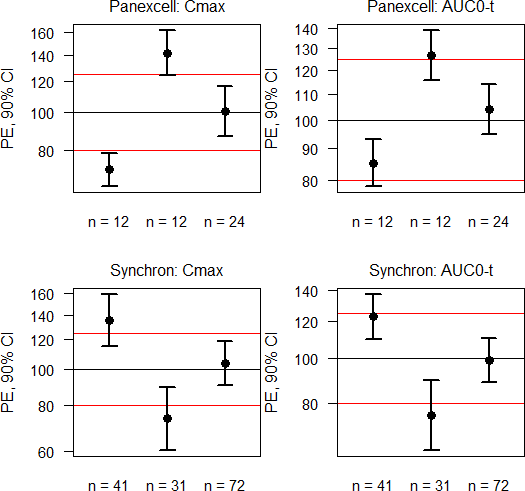## Visualization [GxP / QC / QA]

Hi ElMaestro,

❝ […] it makes very good sense to plot for example the RMSE, CV, SE of diff., or even the width of the CI as function of (cumulated) number of subjects.

If you have all data, of course. A simple visualization using the res data.frame of my previous post:windows(width = 5.5, height = 5.5) op <- par(no.readonly = TRUE) par(mar = c(3, 4, 2, 0), cex.axis = 0.9, cex.main = 1, font.main = 1) split.screen(c(length(CRO), length(metric))) scrn <- 0 for (j in seq_along(CRO)) {   for (k in seq_along(metric)) {     scrn <- scrn + 1     screen(scrn)     plot(c(0.5, length(study)+0.5), rep(100, 2), type = "n",          ylim = range(res$lower[res$CRO == CRO[j] & res$metric == metric[k]], res$upper[res$CRO == CRO[j] & res$metric == metric[k]]),          log = "y", xlab = "", ylab = "PE, 90% CI", axes = FALSE,          main = paste0(CRO[j], ": ", metric[k]))     axis(2, las = 1)     axis(1, at = seq_along(study), tick = FALSE,          labels = paste("n =", res$n[res$CRO == CRO[j] &                                res$metric == metric[k]])) abline(h = c(80, 100, 125), col = c("red", "black", "red")) box() for (l in seq_along(study)) { arrows(x0 = l, y0 = res$lower[res$CRO == CRO[j] & res$metric == metric[k] &                             res$study == study[l]], y1 = res$upper[res$CRO == CRO[j] & res$metric == metric[k] &                             res$study == study[l]], length = 0.08, angle = 90, lwd = 2, code = 3) points(l, res$PE[res$CRO == CRO[j] & res$metric == metric[k] &                        res\$study == study[l]], pch = 19, cex = 1.25)     }   } } close.screen(all = TRUE) par(op)

Dif-tor heh smusma 🖖🏼 Довге життя Україна!Helmut SchützThe quality of responses received is directly proportional to the quality of the question asked. 🚮
Science QuotesIng. Helmut Schütz